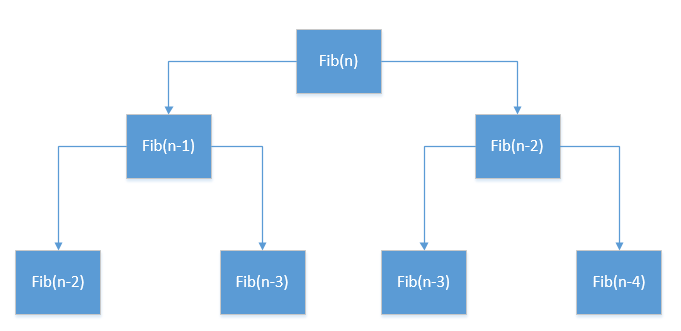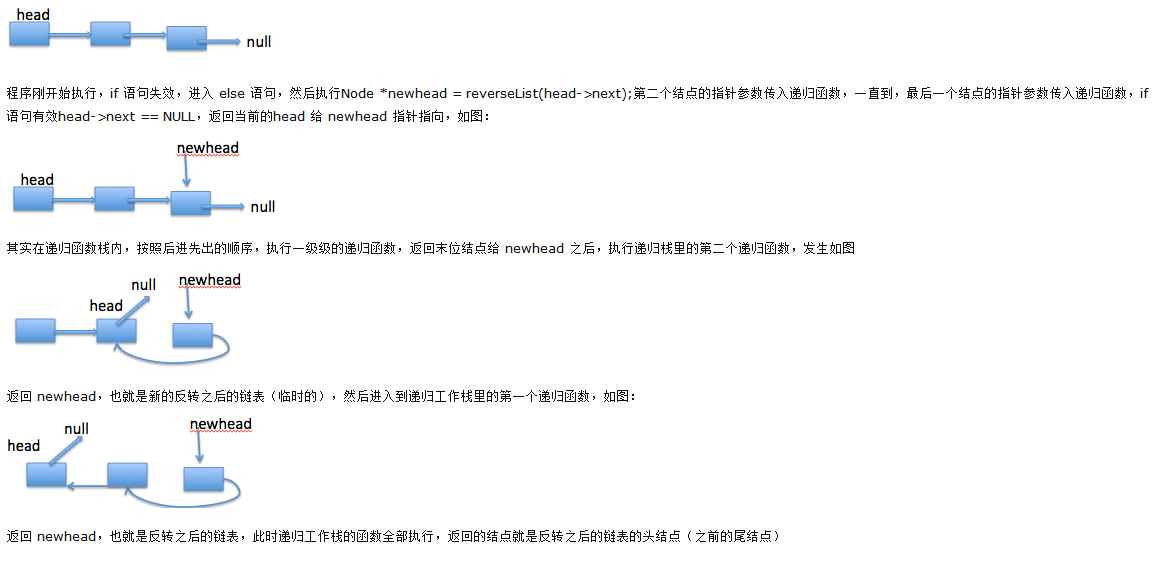• 递归的基本思想递归并不是简单的自己调用自己，也不是简单的交互调用。递归在于把问题分解成规模更小、具有与原来问题相同解法的问题，如二分查找以及求集合的子集问题。这些都是不断的把问题规模变小，新问题与原...
递归的基本思想

递归并不是简单的自己调用自己，也不是简单的交互调用。递归在于把问题分解成规模更小、具有与原来问题相同解法的问题，如二分查找以及求集合的子集问题。这些都是不断的把问题规模变小，新问题与原问题有着相同的解法。但是并不是所有所有可以分解的子问题都能使用递归来求解。一般来说使用递归求解问题需要满足以下的条件：
可以把要解决的问题转化为一个子问题，而这个子问题的解决方法仍与原来的解决方法相同，只是问题的规模变小了。原问题可以通过子问题解决而组合解决。
例如斐波拉契数列问题，一个数列满足 1，1，2，3，5….. 的形式，即当前项为前两项之和的形式，那么则称这个数列为斐波拉契数列。假设现在要求第 n 项数列的值。
则 f(n) 我们可以通过求的 f(n-1),f(n-2) 所得，原问题可以转化为两个子问题，满足条件一。
假设我们现在得到 f(n-1)、f(n-2)。f(n)=f(n-1)+f(n-2), 满足条件二。
原问题可以通过子问题的解决而解决。而 f(1)=1,f(2)=1, 已知，即存在简单情境使得递归退出，满足条件三。所以此问题解法如下

int fib(n){
if(n==1)
return 1;
if(n==2)
return 1;
return fib(n-1)+fib(n-2);}

调用过程 ：通过上面的例子可以总结出递归问题的分析思路

分析问题看是否可以分解成子问题 子问题和原问题之间有何关系 是否有退出的简单条件

例题一：

用递归的实现方式链表的反转问题：

如下图代码如下：

//递归方式
{
//如果链表为空或者链表中只有一个元素
{
}
else
{
//先反转后面的链表，走到链表的末端结点
//再将当前节点设置为后面节点的后续节点

}
}

2、自下而上的递归操作，每次都反转一个链表结点；然后再重复向前推进；
3、结束位置就是第一个调用的函数的位置。

例题二：
给定一个二叉树，找到其最小深度。最小深度是从根节点到最近叶节点的最短路径的节点数。

分析：
1、二叉树是可以分解为子数的形式；
2、关系就是每个数都是通过根连接子树的；
3、跳出位置，就是当根结点不存在时，返回0；当子树的右子树不存在时，计算左子树；同理计算左子树不存在时。
4、分别从左右子树出发，递归计算深度，每存在一个子叶，深度加1，最后取小值。
特别鸣谢！
资料转载： http://blog.csdn.net/yuanmxiang/article/details/52868999 http://www.cnblogs.com/kubixuesheng/p/4394509.html
展开全文• ​// 编程之美1.13 nim(3) 两对石头的游戏 // 一种递归解法 package main import ( "fmt" ) // 两堆石头， 假定 n &lt;= m type pair struct { n, m int } var mapsafe map[pair]bool func main()...
​// 编程之美1.13 nim(3) 两对石头的游戏
//     一种递归解法
package main
import (
"fmt"
)

// 两堆石头， 假定 n <= m
type pair struct {
n, m int
}

var mapsafe map[pair]bool
func main() {
mapsafe = make(map[pair]bool, 100)
nim(17, 24)

// 打印不安全局面
for k, v := range mapsafe {
if !v {
fmt.Println(k)
}
}
}

func nim(n, m int) bool {
if n == m {
return true
}

if n > m {
t := n
n = m
m = t
}

result, ok := mapsafe[pair{n, m}]
if ok {
return result
}

// 若 (i,m) 或 （i, m-n+i)中有不安全局面， 则（n, m）是安全的
for i := 1; i < n; i++ {
if !nim(i, m) || !nim(i, m-n+i) {
mapsafe[pair{n, m}] = true
return true
}
}

// 若 （n, i)中有不安全局面， 则（n, m)是安全的
for i := 1; i < m; i++ {
if !nim(n, i) {
mapsafe[pair{n, m}] = true
return true
}
}

mapsafe[pair{n, m}] = false
return false
}


展开全文golang
• 看了书上的递归算法，想转成非递归算法。模仿前序遍历er
看了书上的递归算法，想转成非递归算法。
模仿前序遍历二叉树的非递归实现。
element结构体的定义见下边的完整代码。
非递归算法：

void ReBuild1(char* pPreOrder, char* pInOrder, int nTreeLen, Node** pRoot) //模仿前序遍历二叉树的非递归算法
{
element s[TREELEN];
int top = -1;
int leftLen;
if(nTreeLen<=0 || !pPreOrder || !pInOrder)
return;
while(top != -1 || nTreeLen>0)
{
while(nTreeLen>0)
{
Node* pTemp = new Node;
pTemp->chValue = *pPreOrder;
pTemp->pLeft = NULL;
pTemp->pRight = NULL;
*pRoot = pTemp;
char* pLeftEnd = pInOrder;
leftLen = 0;
while(*pPreOrder != *pLeftEnd)
{
leftLen++;
if(leftLen>nTreeLen)
return;
pLeftEnd++;
}
top++;
s[top].pPreOrder = pPreOrder;
s[top].pInOrder = pInOrder;
s[top].leftLen = leftLen;
s[top].len = nTreeLen;
s[top].pRoot = pRoot;
pPreOrder++;  //对应ReBuild(pPreOrder+1,pInOrder,leftLen,pRoot->pLeft);
pRoot = &((*pRoot)->pLeft);
nTreeLen = leftLen;
}
if(top != -1)
{
pPreOrder = s[top].pPreOrder;
pInOrder = s[top].pInOrder;
leftLen = s[top].leftLen;
nTreeLen = s[top].len;
pRoot = s[top].pRoot;
top--;
pPreOrder = pPreOrder+leftLen+1; //对应ReBuild(pPreOrder+leftLen+1,pInOrder+leftLen+1,nRightLen,pRoot->pRight);
pInOrder = pInOrder+leftLen+1;
nTreeLen = nTreeLen-leftLen-1;
pRoot = &((*pRoot)->pRight);
}

}
}

把书上递归算法的边界条件稍微改了下。
递归算法：

void ReBuild(char* pPreOrder, char* pInOrder, int nTreeLen, Node* &pRoot) //递归 不带返回值
{
if(nTreeLen <= 0 || !pPreOrder || !pInOrder)
return;
Node* pTemp = new Node;
pTemp->chValue = *pPreOrder;
pTemp->pLeft = NULL;
pTemp->pRight = NULL;
pRoot = pTemp;
char* pLeftEnd = pInOrder;
int leftLen = 0;
while(*pPreOrder != *pLeftEnd)
{
leftLen++;
if(leftLen>nTreeLen)
return;
pLeftEnd++;
}
int nRightLen = nTreeLen-leftLen-1;
ReBuild(pPreOrder+1,pInOrder,leftLen,pRoot->pLeft);
ReBuild(pPreOrder+leftLen+1,pInOrder+leftLen+1,nRightLen,pRoot->pRight);
}

完整的代码：

#include<iostream>
using namespace std;

#define TREELEN 6

struct Node
{
Node* pLeft;
Node* pRight;
char chValue;
};

struct element
{
char* pPreOrder;
char* pInOrder;
int leftLen;
int len;
Node** pRoot;
};

void ReBuild(char* pPreOrder, char* pInOrder, int nTreeLen, Node* &pRoot) //递归 不带返回值
{
if(nTreeLen <= 0 || !pPreOrder || !pInOrder)
return;
Node* pTemp = new Node;
pTemp->chValue = *pPreOrder;
pTemp->pLeft = NULL;
pTemp->pRight = NULL;
pRoot = pTemp;
char* pLeftEnd = pInOrder;
int leftLen = 0;
while(*pPreOrder != *pLeftEnd)
{
leftLen++;
if(leftLen>nTreeLen)
return;
pLeftEnd++;
}
int nRightLen = nTreeLen-leftLen-1;
ReBuild(pPreOrder+1,pInOrder,leftLen,pRoot->pLeft);
ReBuild(pPreOrder+leftLen+1,pInOrder+leftLen+1,nRightLen,pRoot->pRight);
}

void ReBuild1(char* pPreOrder, char* pInOrder, int nTreeLen, Node** pRoot) //模仿前序遍历二叉树的非递归算法
{
element s[TREELEN];
int top = -1;
int leftLen;
if(nTreeLen<=0 || !pPreOrder || !pInOrder)
return;
while(top != -1 || nTreeLen>0)
{
while(nTreeLen>0)
{
Node* pTemp = new Node;
pTemp->chValue = *pPreOrder;
pTemp->pLeft = NULL;
pTemp->pRight = NULL;
*pRoot = pTemp;
char* pLeftEnd = pInOrder;
leftLen = 0;
while(*pPreOrder != *pLeftEnd)
{
leftLen++;
if(leftLen>nTreeLen)
return;
pLeftEnd++;
}
top++;
s[top].pPreOrder = pPreOrder;
s[top].pInOrder = pInOrder;
s[top].leftLen = leftLen;
s[top].len = nTreeLen;
s[top].pRoot = pRoot;
pPreOrder++;  //对应ReBuild(pPreOrder+1,pInOrder,leftLen,pRoot->pLeft);
pRoot = &((*pRoot)->pLeft);
nTreeLen = leftLen;
}
if(top != -1)
{
pPreOrder = s[top].pPreOrder;
pInOrder = s[top].pInOrder;
leftLen = s[top].leftLen;
nTreeLen = s[top].len;
pRoot = s[top].pRoot;
top--;
pPreOrder = pPreOrder+leftLen+1; //对应ReBuild(pPreOrder+leftLen+1,pInOrder+leftLen+1,nRightLen,pRoot->pRight);
pInOrder = pInOrder+leftLen+1;
nTreeLen = nTreeLen-leftLen-1;
pRoot = &((*pRoot)->pRight);
}

}
}

Node* ReBuild2(char* pPreOrder, char* pInOrder, int nTreeLen) //递归 带返回值
{
if(nTreeLen<=0 || !pPreOrder || !pInOrder)
return NULL;
Node* pTemp = new Node;
pTemp->chValue = *pPreOrder;
int leftLen = 0;
char* pEnd = pInOrder;
while(*pPreOrder != *pEnd)
{
leftLen++;
pEnd++;
if(leftLen > nTreeLen)
return NULL;
}
int rightLen = nTreeLen - leftLen - 1;
pTemp->pLeft = ReBuild2(pPreOrder+1,pInOrder,leftLen);
pTemp->pRight = ReBuild2(pPreOrder+leftLen+1,pEnd+1,rightLen);
return pTemp;
}

void PreOrder(Node* root)
{
if(root == NULL) return;
cout<<root->chValue<<endl;
PreOrder(root->pLeft);
PreOrder(root->pRight);
delete root;  //释放内存
}

int main()
{
char preOrder[TREELEN]={'a','b','d','c','e','f'};
char inOrder[TREELEN]={'d','b','a','e','c','f'};
Node* pRoot = NULL;
ReBuild(preOrder,inOrder,TREELEN,pRoot);
PreOrder(pRoot); //释放内存
cout<<"************************"<<endl;
ReBuild1(preOrder,inOrder,TREELEN,&pRoot);
PreOrder(pRoot); //释放内存
cout<<"************************"<<endl;
pRoot = ReBuild2(preOrder,inOrder,TREELEN);
PreOrder(pRoot); //释放内存
system("pause");
return 0;
}


展开全文重建二叉树
• 另外参考了编程之美的写法 一般人的写法 while循环体有2次的比较 而这里第三种写法 while循环体只有1次的比较 虽然两者算法复杂度为O（lgn） 但是常数因子有了极大的提升 建议参考最优的二分查找写法  ...
二分查找  是个经典的问题 但是 考虑到很多边界条件 能在短时间的面试环节  写出个零bug版本 还是需要多code
本人总结了自己写的三个版本 递归与非递归
另外参考了编程之美的写法 一般人的写法 while循环体有2次的比较   而这里第三种写法 while循环体只有1次的比较
虽然两者算法复杂度为O（lgn） 但是常数因子有了极大的提升 建议参考最优的二分查找写法

#include <iostream>

using namespace std;

/** 二分查找
*
* key  待查找元素
* begin 数组起始位置
* end   数组结束位置
* 返回值为待查找的元素在数组位置   若找不到 则返回-1
*
*/
int BinarySearch(int  array[], int begin, int end,int key)
{
while(begin<=end)
{
int mid=begin+(end-begin)/2;
if(array[mid]==key)
{return mid;}
else if (array[mid]>key)
{return BinarySearch(array,begin,mid-1,key);}
else
{return BinarySearch(array,mid+1,end,key);}
}
return -1;
}

int BinarySeachNonRecursive(int  array[], int begin, int end,int key)
{
int mid;
while(begin<=end)
{
mid=begin+(end-begin)/2;
if(array[mid]==key)
{return  mid;}
else if (array[mid]>key)
{end=mid-1;}
else
{begin=mid+1;}

}
return -1;
}

// 最优二分查找写法
int BinarySeachOptimal(int  array[], int begin, int end,int key)
{
int mid;
while(begin<end-1)
{
mid=begin+(end-begin)/2;   //防止溢出
if(array[mid]<=key)
{
begin=mid;
}
else{
end=mid;
}
}

if(array[end]==key)
{
return end;
}
if(array[begin]==key)
{
return mid;
}
return -1;
}

int main()
{

int num;
cin >> num;
int array[num];
for(int i=0;i<num;i++)
{
cin >> array[i];
}
int key;
cin >>  key;
//int index=BinarySearch(array,0,num-1,key);
//int index=BinarySeachNonRecursive(array,0,num-1,key);
int index=BinarySeachOptimal(array,0,num-1,key);
if(index>=0)
{
cout << "the index of "<< key << " is "<< index<<endl;
}
else{
}
//cout << "Hello world!" << endl;
return 0;
}

展开全文c++ 二分查找 算法
• 此题来着编程之美 //以下用 #include #include using namespace std; char num_str = { "", "", "abc", "def", "ghi", "jkl", "mno", "pqrs", "tuv", "wxyz" }; char * num_to_str(char *...
• 斐波那契数列的递推关系式 ...fr=aladdin 递归代码展示： int Fibonacci(int n){ if (n&amp;lt;=0) { return 0; } else if (n==1){ return 1; } else{ return Fibonacci(n-1)+Fibon...斐波那契数列
• ## 编程之美_004硬币找零,递归算法

千次阅读 多人点赞 2013-01-09 10:07:42
// 硬币找零 递归算法 public class Test_001 { // 硬币的类型 private static int[] money = { 10, 5, 2, 1 }; // 每个硬币对应的数量 private static int[] tote = new int[money.length]; pub硬币找零
• 先求当前节点之前，先得求左右子节点为根的子树的深度，用递归肯定是很方便的方法，不过不用递归也是可以的。 二叉树的层次遍历会从根节点往子节点逐层遍历所有节点，把这些节点按顺序存储到双向链表中，再反向...
• 这里给出书中扩展问题提出的非递归解法。就像书中总结的时候给出的叶先生博客中关于本题的解法，这里同样不需要在节点中嵌入nMaxLeft、nMaxRight等额外数据。 主要原理是基于非递归后续遍历二叉树。额外需要两个栈...
• 编程之美中的最大公约数问题，下面通过代码依次实现. 解法一、欧几里得的辗转相除法 int gcd(int x,int y){ return (!y)?x:gcd(y,x%y); }解法二、辗转相减法 int gcd(int x,int y){ if(x) return gcd(y,x); ...
• 斐波那契数的计算公式是F(n)=F(n-1)+F(n-2),F(0)=F(1)=1 ...任何递推的数学公式都可以直接翻译为递归的算法，于是递归的 斐波那契数计算方法是这样的：   int fib(int n){算法 float struct null
• 编程之美笔记---如何计算24点（递归和动态规划两种方法）
• The basic program is to get the height of a binary tree. We could use BFS to realize that. So the result is :  #include #include #include using namespace std; class TreeNode { ... int
• 下面先给出对于某个节点的前序、中序、后序遍历的定义： 1、前序遍历： 先访问该节点，然后在递归遍历其左子树，然后再访问其右子树 2、中序遍历： 先递归遍历其左子树，然后访问该节点，然后再递归遍历其右子树 3...tree null struct 数据结构
• #include "stdafx.h" unsigned int F(unsigned int n) { if(n==0) return 0; if(n==1) return 1; if(n>=2) return F(n-1)+F(n-2);...递归实现的代码很简洁,很优美...但是递归的效率比不上迭代,大家可以比较一下.
• 用c++实现了编程之美这本书中的买书问题。分别用递归和非递归两种方式是实现，当购买书的数量为10+10+10+10+10时，递归无法运行出结果。
• 问题： 对如非全键盘的手机上的数字，每个数字都对应一些字母，比如2对应ABC，3对应DEF.........，8对应TUV，9对应WXYZ，要求对...以下是非递归的程序，比较有技巧性。 // File : print_phone_word.cc // Date : 201date file 手机
• 这是我在编程之美上找的题目，感觉自己有自己的想法，对于书上的解法目前还没有仔细看。 题目 思路 我一次看到这种题就是递归函数去解决这种考虑情况的问题，当然我不会用递归...面试题 c++ dp
• 编程之美上面已经说的很清楚了，使用蛮力法时间复杂度为O（n*n），使用分治递归时间复杂度为O（n*log n）。以下是代码实现： #include #include #include #include using namespace std; typedef tuple dpoint; /...C++ 寻找最近点对
• 递归是一种应用非常广泛的算法（或编程技巧）。一般来说，递归需要有边界条件、递归前进段和递归返回段。当边界条件不满足时，递归前进；当边界条件满足时，递归返回。 举个例子：你带着女朋友去看电影，女朋友问：...
• 最近接触erlang，一直在看文档和源码，终于自己尝试写点简单代码，却意外发现erlang里的编程之美。一直写C++，用惯了while，for这些循环语句。而erlang不支持变量重复赋值，因而也不支持循环语句。erlang能使用的...erlang list
• 编程之美 2.9 斐波那契(Fibonacci)数列斐波那契的递归表达式如下 F(n)=F(n-1)+F(n-2) n>=2 F(1)=1 F(0)=0 书中提到了三中解决方法Fibonacci 斐波那契数列 java
• 1.常规递归 2.进行二次矩阵分解，然后利用求指数的方法，分解为按照指数的二进制来求解算法
• 我个人的 PHP 编程经验中，递归调用常常与静态变量使用。静态变量的含义可以参考 PHP 手册。希望下面的代码，会更有利于对递归以及静态变量的理解   header("Content-type: text/plain"); function ...php 重构
• 递归也不见得就增加存储空间，许多涉及到树和图的递归算法中，其总的空间复杂度还是图或树的节点数，在自己的编程之美P253中有这方面的笔记。 动态规划是一种思想，可以用递归实现，之前在算法导论中说动态规划的...
• 斐波那契数列是我们在学习C语言的时候，在递归那一章的经典实例，当然，还会有汉诺塔的例子。 这个问题时这样定义的： 0 (x  f(x) = 1 (x == 1) f(x - 1) + f(x - 2) (x > 1) 看到这个递推公式后，...c语言 typedef 算法
• 如：前序：a b d c e f中序：d b a e c f思路：根据前序，找出根节点a，然后根据中序，可以找出根节点a的左右子树，然后递归求a的左子树前序b d跟中序d b，a的右子树前序c e f跟中序e c fpackage suda.alex.chapter3...遍历
• [读书笔记]编程之美（一)不得不说编程之美是一本很有意思的书，里面的各式各样新奇的问题，总是可以通过课上讲的简单的问题来解决，对于训练自己的思维的确有很大的好处。一般解决复杂的问题，我们总是可以通过：1、...
• 我们在上一章的复习讲座中已经提到了Scheme中的递归调用。我们熟悉C/C++或Java等命令式编程语言的朋友对递归应该不陌生吧，呵呵。我们本讲将介绍一下Scheme中的递归调用。scheme 语言 java 存储
• 同时找到最大值和最小值——编程之美    给定一个数组，我们可以同时找到其中的最大数和最小数吗？要求时间复杂度尽可能的小。 编程之美上面提供了三个思路，我把它们都实现了，并做一些讲解补充。  ...时间复杂度...